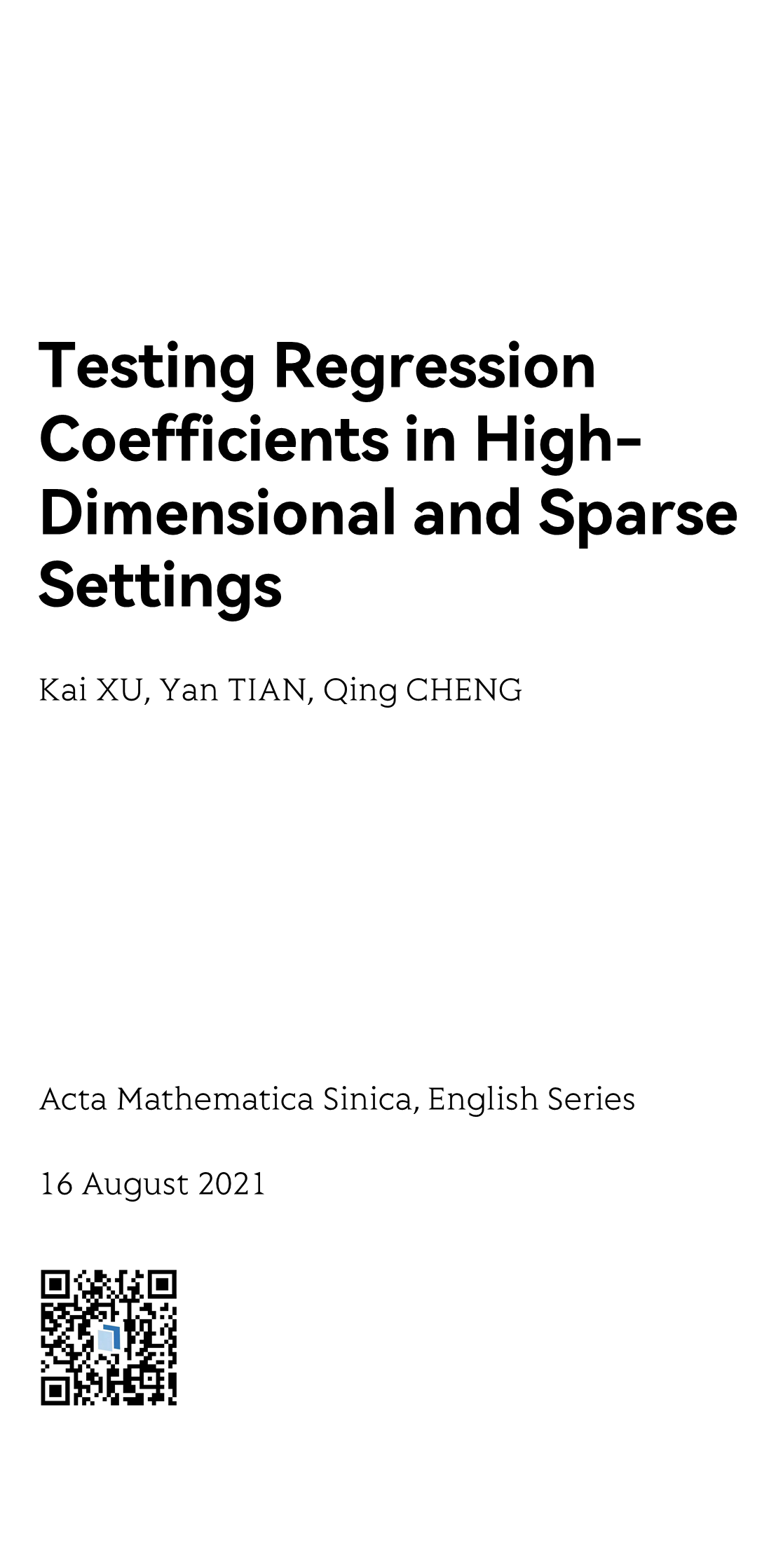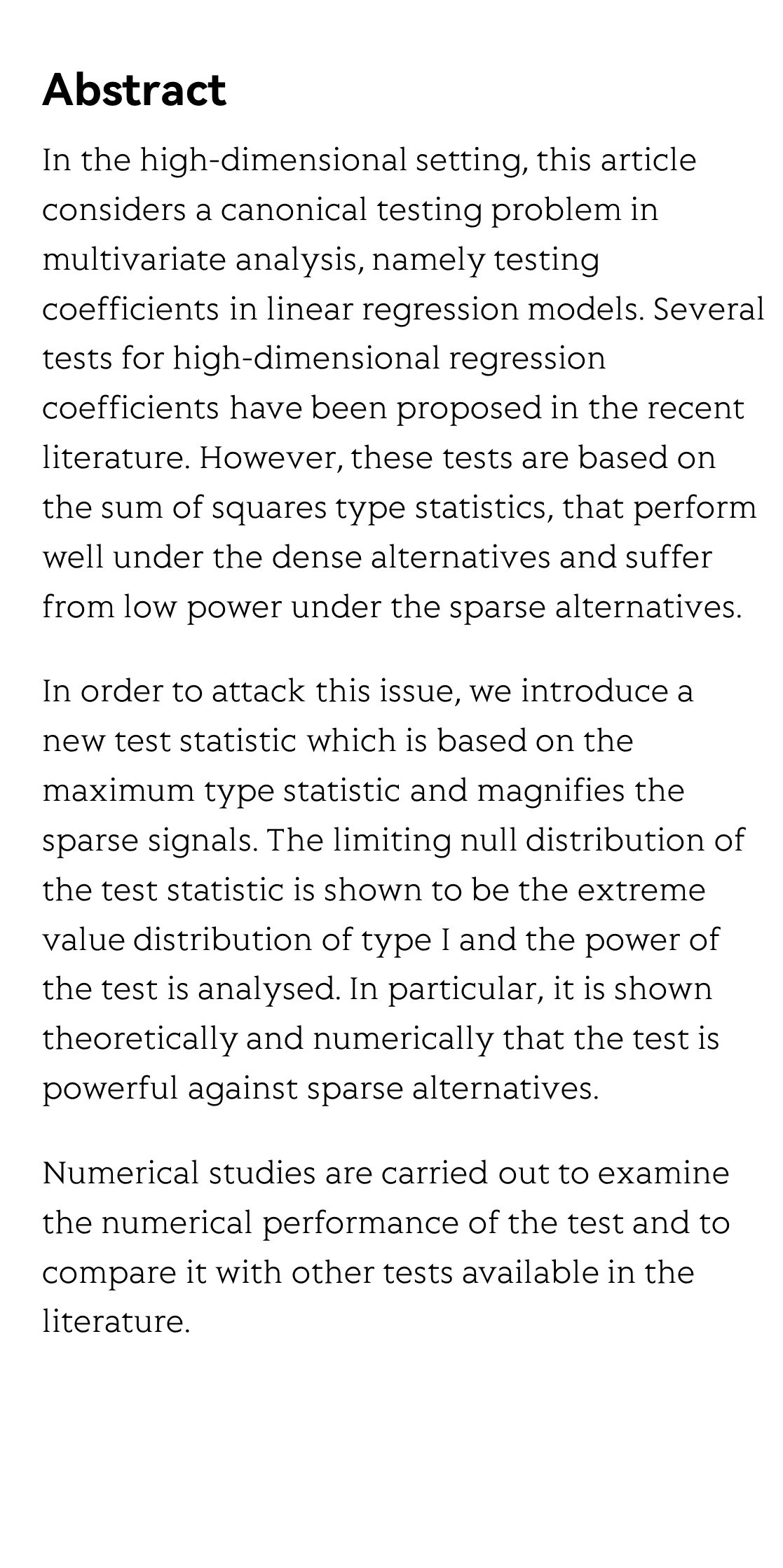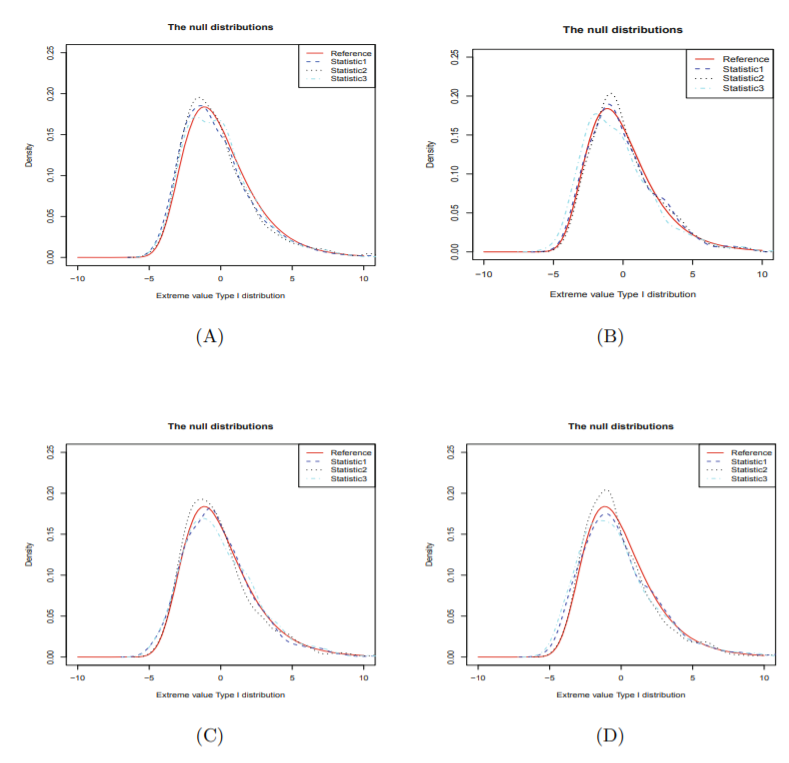MMMYYYYTesting Regression Coefficients in High-Dimensional and Sparse Settings

고차원 및 희소 설정에서 회귀 계수 테스트하기
Prueba de coeficientes de regresión en entornos de alta dimensión y dispersos
Test des coefficients de régression dans des paramètres de grande dimension et clairsemés
Тестирование коэффициентов регрессии в многомерных и разреженных настройках
Kai XU 许凯 ¹, Yan TIAN 田艳 ¹, Qing CHENG ²
¹ School of Mathematics and Statistics, Anhui Normal University, Wuhu 241002, P. R. China

² Center for Quantitative Medicine, Duke-NUS Medical School, National University of Singapore, Singapore 169856
Acta Mathematica Sinica, English Series, 15 October 2021Abstract

In the high-dimensional setting, this article considers a canonical testing problem in multivariate analysis, namely testing coefficients in linear regression models. Several tests for high-dimensional regression coefficients have been proposed in the recent literature. However, these tests are based on the sum of squares type statistics, that perform well under the dense alternatives and suffer from low power under the sparse alternatives.

In order to attack this issue, we introduce a new test statistic which is based on the maximum type statistic and magnifies the sparse signals. The limiting null distribution of the test statistic is shown to be the extreme value distribution of type I and the power of the test is analysed. In particular, it is shown theoretically and numerically that the test is powerful against sparse alternatives.

Numerical studies are carried out to examine the numerical performance of the test and to compare it with other tests available in the literature.Reviews and Discussions